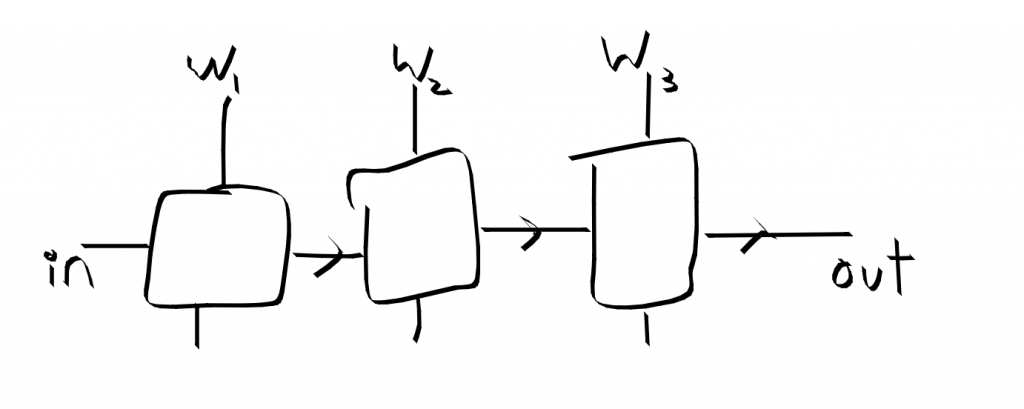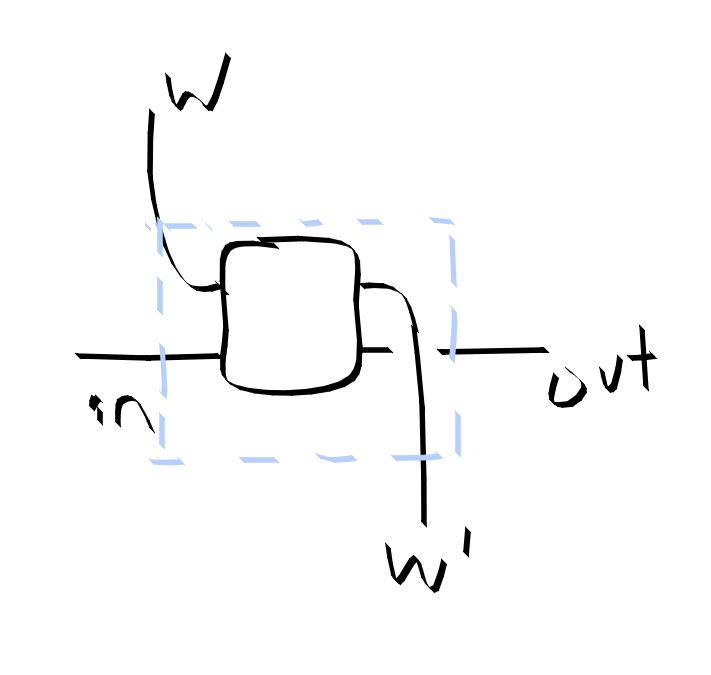I wrote a while back how you can make a pretty nice DSL for reverse mode differentiation based on the same type as Lens. I’d heard some interesting rumblings on the internet around these ideas and so was revisiting them.

http://www.philipzucker.com/reverse-mode-differentiation-is-kind-of-like-a-lens-ii/


type Lens s t a b = s -> (a, b -> t)
type AD x dx y dy = x -> (y, dy -> dx)


Composition is defined identically for reverse mode just as it is for lens.The forward computation shares info with the backwards differential propagation, which corresponds to a transposed Jacobian

After chewing on it a while, I realized this really isn’t that exotic. How it works is that you store the reverse mode computation graph, and all necessary saved data from the forward pass in the closure of the (dy -> dx). I also have a suspicion that if you defunctionalized this construction, you’d get the Wengert tape formulation of reverse mode ad.

Second, Lens is just a nice structure for bidirectional computation, with one forward pass and one backward pass which may or may not be getting/setting. There are other examples for using it like this.

It is also pretty similar to the standard “dual number” form type FAD x dx y dy = (x,dx)->(y,dy) for forward mode AD. We can bring the two closer by a CPS/Yoneda transformation and then some rearrangement.


x -> (y, dy -> dx)
==>  x -> (y, forall s. (dx -> s) -> (dy -> s))
==>  forall s. (x, dx -> s) -> (y, dx -> s)


and meet it in the middle with


(x,dx) -> (y,dy)
==> forall s. (x, s -> dx) -> (y, s -> dy)


I ended the previous post somewhat unsatisfied by how ungainly writing that neural network example was, and I called for Conal Elliot’s compiling to categories plugin as a possible solution. The trouble is piping the weights all over the place. This piping is very frustrating in point-free form, especially when you know it’d be so trivial pointful. While the inputs and outputs of layers of the network compose nicely (you no longer need to know about the internal computations), the weights do not. As we get more and more layers, we get more and more weights. The weights are in some sense not as compositional as the inputs and outputs of the layers, or compose in a different way that you need to maintain access to.

I thought of a very slight conceptual twist that may help.

The idea is we keep the weights out to the side in their own little type parameter slots. Then we define composition such that it composes input/outputs while tupling the weights. Basically we throw the repetitive complexity appearing in piping the weights around into the definition of composition itself.

These operations are easily seen as 2 dimensional diagrams.Three layers composed, exposing the weights from all layersThe 2-D arrow things can be built out of the 1-d arrows of the original basic AD lens by bending the weights up and down. Ultimately they are describing the same thing

Here’s the core reverse lens ad combinators


import Control.Arrow ((***))

type Lens'' a b = a -> (b, b -> a)

comp :: (b -> (c, (c -> b))) -> (a -> (b, (b -> a))) -> (a -> (c, (c -> a)))
comp f g x = let (b, dg) = g x in
let (c, df) = f b in
(c, dg . df)

id' :: Lens'' a a
id' x = (x, id)

relu' :: (Ord a, Num a) => Lens'' a a
relu' = \x -> (frelu x, brelu x) where
frelu x | x > 0 = x
| otherwise = 0
brelu x dy | x > 0 = dy
| otherwise = 0

add' :: Num a => Lens'' (a,a) a
add' = \(x,y) -> (x + y, \ds -> (ds, ds))

dup' :: Num a => Lens'' a (a,a)
dup' = \x -> ((x,x), \(dx,dy) -> dx + dy)

sub' :: Num a => Lens'' (a,a) a
sub' = \(x,y) -> (x - y, \ds -> (ds, -ds))

mul' :: Num a => Lens'' (a,a) a
mul' = \(x,y) -> (x * y, \dz -> (dz * y, x * dz))

recip' :: Fractional a => Lens'' a a
recip' = \x-> (recip x, \ds -> - ds / (x * x))

div' :: Fractional a => Lens'' (a,a) a
div' = (\(x,y) -> (x / y, \d -> (d/y,-x*d/(y * y))))

sin' :: Floating a => Lens'' a a
sin' = \x -> (sin x, \dx -> dx * (cos x))

cos' :: Floating a => Lens'' a a
cos' = \x -> (cos x, \dx -> -dx * (sin x))

pow' :: Num a => Integer -> Lens'' a a
pow' n = \x -> (x ^ n, \dx -> (fromInteger n) * dx * x ^ (n-1))

--cmul :: Num a => a -> Lens' a a
--cmul c = lens (* c) (\x -> \dx -> c * dx)

exp' :: Floating a => Lens'' a a
exp' = \x -> let ex = exp x in
(ex, \dx -> dx * ex)

fst' :: Num b => Lens'' (a,b) a
fst' = (\(a,b) -> (a, \ds -> (ds, 0)))

snd' :: Num a => Lens'' (a,b) b
snd' = (\(a,b) -> (b, \ds -> (0, ds)))

-- some monoidal combinators
swap' :: Lens'' (a,b) (b,a)
swap' = (\(a,b) -> ((b,a), \(db,da) -> (da, db)))

assoc' :: Lens'' ((a,b),c) (a,(b,c))
assoc' = \((a,b),c) -> ((a,(b,c)), \(da,(db,dc)) -> ((da,db),dc))

assoc'' :: Lens'' (a,(b,c)) ((a,b),c)
assoc'' = \(a,(b,c)) -> (((a,b),c), \((da,db),dc)->  (da,(db,dc)))

par' :: Lens'' a b -> Lens'' c d -> Lens'' (a,c) (b,d)
par' l1 l2 = l3 where
l3 (a,c) = let (b , j1) = l1 a in
let (d, j2) = l2 c in
((b,d) , j1 *** j2)
first' :: Lens'' a b -> Lens'' (a, c) (b, c)
first' l = par' l id'

second' :: Lens'' a b -> Lens'' (c, a) (c, b)
second' l = par' id' l

labsorb :: Lens'' ((),a) a
labsorb (_,a) = (a, \a' -> ((),a'))

labsorb' :: Lens'' a ((),a)
labsorb' a = (((),a), \(_,a') -> a')

rabsorb :: Lens'' (a,()) a
rabsorb = comp labsorb swap'


And here are the two dimensional combinators. I tried to write them point-free in terms of the combinators above to demonstrate that there is no monkey business going on. We


type WAD' w w' a b = Lens'' (w,a) (w',b)
type WAD'' w a b = WAD' w () a b -- terminate the weights for a closed network
{- For any monoidal category we can construct this composition? -}
-- horizontal composition
hcompose :: forall w w' w'' w''' a b c. WAD' w' w'' b c -> WAD' w w''' a b -> WAD' (w',w) (w'',w''') a c
hcompose f g = comp f' g' where
f' :: Lens'' ((w',r),b) ((w'',r),c)
f' = (first' swap') comp assoc'' comp (par' id' f) comp assoc' comp  (first' swap')
g' :: Lens'' ((r,w),a) ((r,w'''),b)
g' = assoc'' comp (par' id' g) comp assoc'

rotate :: WAD' w w' a b -> WAD' a b w w'
rotate f = swap' comp f comp swap'

-- vertical composition of weights
vcompose :: WAD' w'  w'' c d -> WAD' w w' a b -> WAD' w w'' (c, a) (d, b)
vcompose f g = rotate (hcompose (rotate f)  (rotate g) )

-- a double par.
diagpar :: forall w w' a b w'' w''' c d. WAD' w  w' a b -> WAD' w'' w''' c d
-> WAD' (w,w'') (w',w''') (a, c) (b, d)
diagpar f g = t' comp (par' f g) comp t where
t :: Lens'' ((w,w''),(a,c)) ((w,a), (w'',c)) -- yikes. just rearrangements.
t =  assoc'' comp (second' ((second' swap') comp assoc' comp swap')) comp assoc'
t' :: Lens'' ((w',b), (w''',d)) ((w',w'''),(b,d)) -- the tranpose of t
t' =  assoc'' comp (second'  ( swap'  comp assoc'' comp (second' swap')))  comp assoc'

id''' :: WAD' () () a a
id''' = id'

-- rotate:: WAD' w a a w
-- rotate = swap'

liftIO :: Lens'' a b -> WAD' w w a b
liftIO = second'

liftW :: Lens'' w w' -> WAD' w w' a a
liftW = first'

wassoc' = liftW assoc'
wassoc'' = liftW assoc''

labsorb'' :: WAD' ((),w) w a a
labsorb'' = first' labsorb

labsorb''' :: WAD' w ((),w) a a
labsorb''' = first' labsorb'

wswap' :: WAD' (w,w') (w',w) a a
wswap' = first' swap'
-- and so on we can lift all combinators


I wonder if this is actually nice?

I asked around and it seems like this idea may be what davidad is talking about when he refers to dioptics

http://events.cs.bham.ac.uk/syco/strings3-syco5/slides/dalrymple.pdf

Perhaps this will initiate a convo.

Edit: He confirms that what I’m doing appears to be a dioptic. Also he gave a better link http://events.cs.bham.ac.uk/syco/strings3-syco5/papers/dalrymple.pdf

He is up to some interesting diagrams